• 设有文法G[S]：S→SaF | F F→FbP | P P→c | d (1) 构造G[S]的算符优先关系表 (2) 分别给出cadbdac# 和 dbcabc# 的分析过程编译原理
• 1、 实验目的：采用算符优先分析法对表达式进行分析，掌握算符优先分析法的基本原理和实现过程。 2、 实验要求： 文法：无二义性的算术表达式的文法 （1）把词法分析作为语法分析的子程序实现（5分） （2）独立的...算符优先分析法
• 编译原理实验3-算符优先分析法#include#include#include#include#define SIZE 128char priority; //算符优先关系表数组char input[SIZE]; //存放输入的要进行分析的句子char remain[SIZE]; //存放剩余串char ...

编译原理实验3-算符优先分析法

#include

#include

#include

#include

#define SIZE 128

char priority; //算符优先关系表数组

char input[SIZE]; //存放输入的要进行分析的句子

char remain[SIZE]; //存放剩余串

char AnalyseStack[SIZE]; //分析栈

void analyse();

int testchar(char x); //判断字符X在算符优先关系表中的位置

void remainString(); //移进时处理剩余字符串，即去掉剩余字符串第一个字符

int k;

void init()//构造算符优先关系表，并将其存入数组中

{

priority='>';

priority='>';

priority='

priority='

priority='>';

priority='>';

priority='>';

priority='>';

priority='$';//无优先关系的用$表示

priority='$'; priority='>'; priority='>'; priority=' priority=' priority=' priority=' priority='='; priority='$';

priority='>';

priority='>';

priority='$'; priority='$';

priority='>';

priority='>';

priority='

priority='

priority='

priority='

priority='$'; priority='='; } void analyse()//对所输入的句子进行算符优先分析过程的函数 { int i,j,f,z,z1,n,n1,z2,n2; int count=0;//操作的步骤数 char a; //用于存放正在分析的字符 char p,Q,p1,p2; f=strlen(input); //测出数组的长度 for(i=0;i<=f;i++) { a=input[i]; if(i==0) remainString(); if(AnalyseStack[k]=='+'||AnalyseStack[k]=='*'||AnalyseStack[k]=='i' ||AnalyseStack[k]=='('||AnalyseStack[k]==')'||AnalyseStack[k]=='#') j=k; else j=k-1; z=testchar(AnalyseStack[j]);//从优先关系表中查出s[j]和a的优先关系 if(a=='+'||a=='*'||a=='i'||a=='('||a==')'||a=='#') n=testchar(a); else //如果句子含有不是终结符集合里的其它字符，不合法 { printf("错误！该句子不是该文法的合法句子！\n"); break; } p=priority[z][n]; if(p=='$')

{

printf("错误！该句子不是该文法的合法句子！\n");

return;

}

if(p=='>')

{ for( ; ; )

{

Q=AnalyseStack[j];

if(AnalyseStack[j-1]=='+'||AnalyseStack[j-1]=='*'||AnalyseStack[j-1]=='i'

||AnalyseStack[j-1]=='('||AnalyseStack[j-1]==')'||AnalyseStack[j-1]=='#')

j=j-1;

else

j=j-2;

z1=testchar(AnalyseStack[j]);

n1=testchar(Q);

p1=priority[z1][n1];

if(p1=='

{

count++;

printf("(%d) %s\t%10c\t%5c%17s\t 归约\n",count,AnalyseStack,p,a,remain);

k=j+1;

i--;

AnalyseStack[k]='N';

int r,r1;

r=strlen(AnalyseStack);

for(r1=k+1;r1

AnalyseStack[r1]='\0';

break;

}

else

continue;

}

}

else

{

if(p=='

{

count++;

printf("(%d) %s\t%10c\t%5c%17s\t 移进\n",count,AnalyseStack,p,a,remain);

k=k+1;

AnalyseStack[k]=a;

remainString();

}

else

{

if(p=='=')

{

z2=testchar(AnalyseStack[j]);

n2=testchar('#');

p2=priority[z2][n2];

if(p2=='=')

{

count++;

printf("(%d) %s\t%10c\t%5c%17s\t 接受\n",count,AnalyseStack,p,a,remain);

printf("该句子是该文法的合法句子。\n");

break;

}

else

{

count++;

printf("(%d) %s\t%10c\t%5c%17s\t 移进\n",count,AnalyseStack,p,a,remain);

k=k+1;

AnalyseStack[k]=a;

remainString();

}

}

else

{

printf("错误！该句子不是该文法的合法句子！\n");

break;

}

}

}

}

}

int testchar(char x)

{

int m;

if(x=='+')

m=0;

if(x=='*')

m=1;

if(x=='i')

m=2;

if(x=='(')

m=3;

if(x==')')

m=4;

if(x=='#')

m=5;

return m;

}

void remainString()

{

int i,j;

i=strlen(remain);

for(j=0;j

remain[j]=remain[j+1];

remain[i-1]='\0';

}

int main()

{

init();

printf("请输入要进行分析的句子(以#号结束输入):\n");

gets(input);//将输入的字符串存到数组中

printf("步骤 栈 优先关系 当前符号 剩余输入串 移进或归约\n");

k=0;

AnalyseStack[k]='#';

AnalyseStack[k+1]='\0';

int length,i; //初始化剩余字符串数组为输入串

length=strlen(input);//

for(i=0;i

remain[i]=input[i];

remain[i]='\0';

analyse();//对所输入的句子进行算符优先分析过程的函数

return 0;

}

展开全文• 自下而上的分析法——算符优先分析法

万次阅读 多人点赞 2018-05-13 19:19:42
算符优先分析法(Operator Precedence Parse)是仿效四则运算的计算过程而构造的一种语法分析方法。算符优先分析法的关键是比较两个相继出现的终结符的优先级而决定应采取的动作。  优点：简单，有效，适合表达式...

概述

算符优先分析法(Operator Precedence Parse)是仿效四则运算的计算过程而构造的一种语法分析方法。算符优先分析法的关键是比较两个相继出现的终结符的优先级而决定应采取的动作。

优点：简单，有效，适合表达式的分析。

缺点：只适合于算符优先文法，是一个不大的文法类。

名词解释

定义：

短语：设有文法G，S是开始符号，设abd是G的一个句型，若有SÞabd且AÞb则称b是句型abd关于A的短语

直接短语：在上面定义中，如果A直接推出b,即AÞb，则称b是句型abd关于A®b的直接短语。

句柄：一个句型的最左直接短语称为句柄。

素短语：文法G某句型的一个短语是素短语，当且仅当它至少含有一个终结符，且除它自身之外不再含更小的素短语。

最左素短语：在具有多个素短语的句型中处于最左边的那个素短语。

求法：

短语：从根结点出发一层一层地找出所有非叶子结点的非终结符，每一个非终结符延伸下去的所有叶子结点从左到右排列起来就是一个短语。

直接短语：找出所有仅有两代的子树，并将它的所有叶子从左到右排列起来就是一个直接短语。

句柄：从直接短语集合中找出最左边的短语。

素短语：从短语集合中找出所有含有终结符的短语，然后选出除它自身之外不再含更小的素短语（这个小的概念是集合中没有被包含的元素，如有两个短语aAA和aAAA，aAA含于aAAA，所以aAA比aAAA小）

最左素短语：从素短语集合中找出最左边的素短语。

FIRSTVT集和LASTVT集

FIRSTVT集

定义：FIRSTVT(P)={a|P=>a…,或P=>Qa…,a属于VT,Q 属于VN}

求法

若P→a…或P→Qa…, 则a属于FIRSTVT(P);

若P→Q…, 则FIRSTVT(Q)含于FIRSTVT(P);

直至FIRSTVT(P)不再增大。

LASTVT集

定义：LASTVT(P)={a|P=>...a,或P=>…aQ,a含于VT,Q 含于VN}

求法

若P→...a或P→…aQ, 则a属于LASTVT(P);

若P→...Q, 则LASTVT(Q)含于LASTVT(P);

直至LASTVT(P)不再增大。

构造算符优先关系表

以以下文法为例：

E→E+T|T

T→T*F|F

F→(E)|i

终结符之间的优先关系

对算符文法G,  a,b属于VT 定义

(1)a=b:  G中有P→. . .ab. . .或P→. . .aQb. . .

(2)a<b:  G中有P→. . .aQ. . .且Q=>b…或Q=>Rb...

(3)a>b:  G中有P→. . .Qb. . . 且Q=>. ..a或Q=>…aR

算符优先关系表的构造

(1)  在文法中添加E→#E#。

(2)  求出FIRSTVT和LASTVT集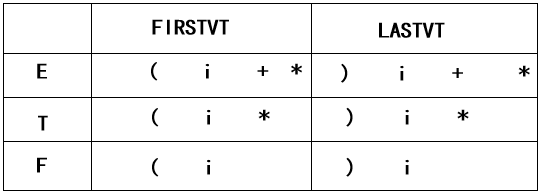(3)  找出所有终结符，并画出关系表的结构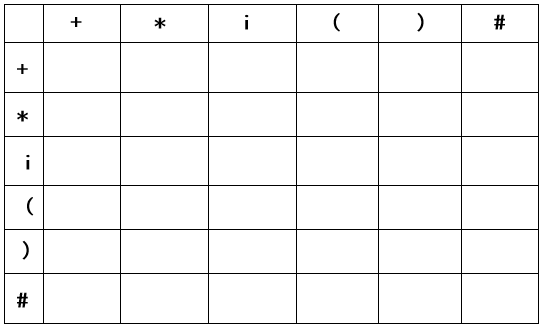(4)  从文法中找出形为aQb（终结符+非终结符+终结符）和ab（终结符+终结符）的部分，本例中为(E)和#E#，然后在(和)与#和#相应的表格填=。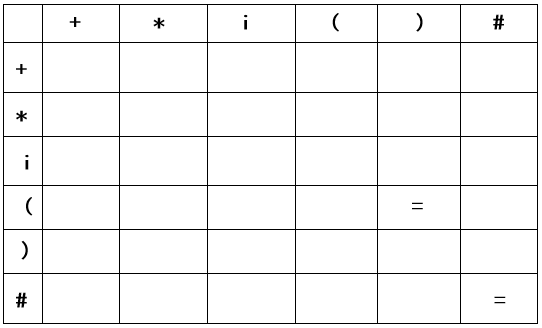(5)  从文法中找出形为aQ（终结符+非终结符）的部分，a与Q的FIRSTVT集合中每一个元素在表格中的交叉点填小于号。

i.找出形为aQ的部分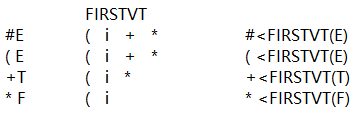ii.填小于号（终结符为竖排，非终结符中的元素为横排，以横排为基准填符号）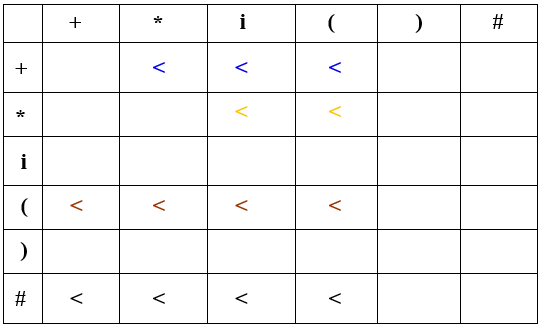(6)  从文法中找出形为Qa（非终结符+终结符）的部分， Q的LASTVT集合中每一个元素与a在表格中的交叉点填大于号。
i.找出形如Qa的部分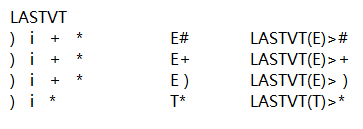ii.填大于号（非终结符中的元素为横排，终结符中的元素为竖排，以竖排为基准填符号）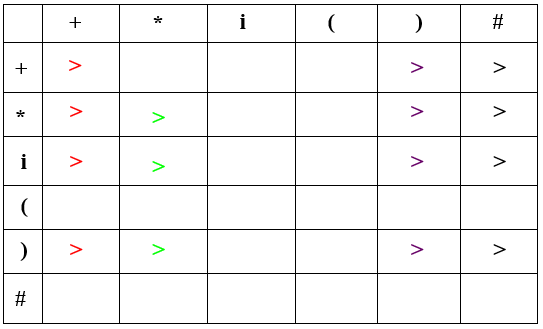(7)  合并后的结果为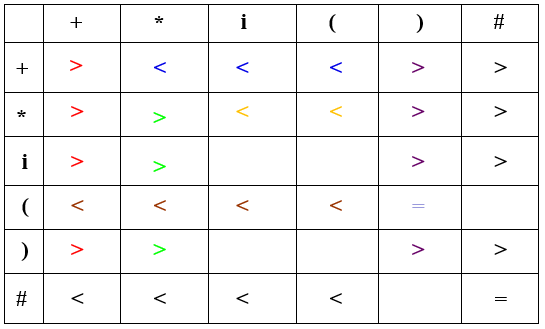从上表可知：

(1)相同终结符之间的优先关系未必是=

(2)有a<b，未必有b>a

(3)a、b之间未必一定有优先关系

故=、<、>不同于关系运算符“等于”、“小于”、“大于”

展开全文编译原理 算符优先分析法 算符优先关系表
• 算符优先分析法 C++ 编译原理 运行环境：Visual Studio 2005 #include "SStack.h" #include #include using namespace std; class Functor { private : char ** table; string ** production; string prog;//...
• 算符优先分析法

前言

参考课上PPT内容。 该学习笔记目前仅打算个人使用。
后续会进一步整理，包括添加笔记内容，标明参考资料。

更新中。。。

参考资料

1. 《编译技术》
2. 邵老师PPT

跳过目录

一、算符优先分析法

算符优先分析（Operator-Precedence Parsing）

• 这是一种经典的自底向上分析法，简单直观，并被广泛使用。

• 开始主要是对表达式的分析，现在已不限于此，可以用于一大类上下文无关文法。

• 称为算符优先分析是因为这种方法是仿效算术式的四则运算而建立起来的。
做算术式的四则运算时，为了保证计算结果和过程的唯一性，规定了一个统一的四则运算法则，确定运算符之间的优先关系。

例：运算法则：

1. 乘除的优先级大于加减；
2. 同优先级的运算符左大于右；
3. 括号内的优先级大于括号外。

于是 4 + 8 - 6 / 2 × 3 运算过程和结果唯一。

思考：4 + 8 - 6 / 2 × 3 第一步进行的运算是哪种运算？

答案：是 + 而非 /，因为读入顺序是自左向右顺序读入的。

1、特点

仿效四则运算过程，预先规定相邻终结符之间的优先关系，然后利用这种优先关系来确定句型的“句柄”，并进行归约。

2、分析器结构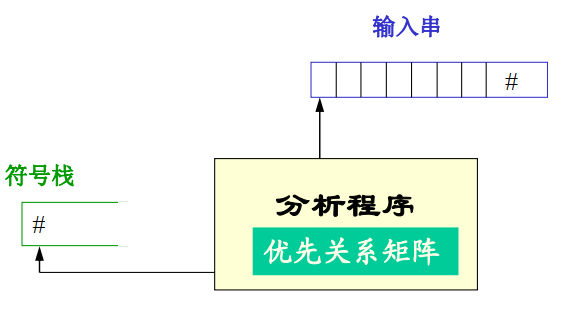3、优先关系

设 a， b 为可能相邻的终结符

• a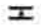b：a的优先级等于b
a ⋖ b：a的优先级小于b
a ⋗ b：a的优先级大于b

例：G[E]

E → E + E | E * E | ( E ) | i
Vt = { + , * , ( , ) , i }

这是一个二义文法，要用算符优先法分析由该文法确定的语言句子。 如： i + i * i

1、确定终结符之间的优先关系

优先关系矩阵（这里先直接给出，后续会学习推导优先关系矩阵）：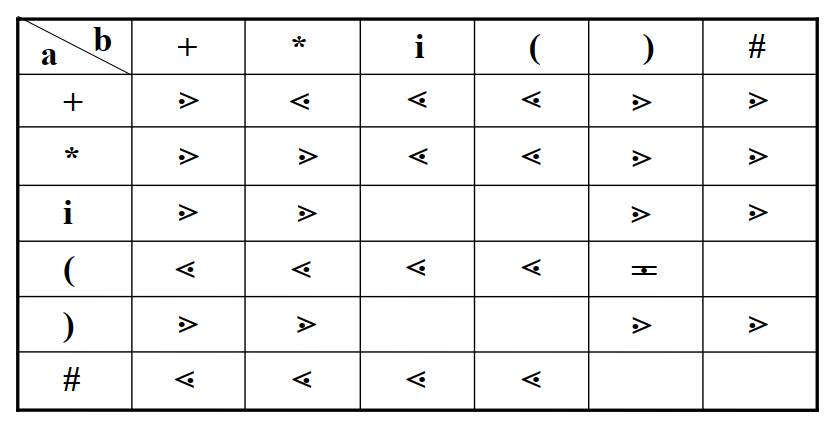矩阵元素空白处表示这两个终结符不能相邻，故没有优先关系。（出现相邻就报错）

2、分析过程

算法：

栈顶项（或次栈顶项） 终结符的优先级大于栈外的终结符的优先级，则进行归约，否则移进。

这里用<、>、= 来代替⋖、⋗、表示

E → E + E | E * E | ( E ) | i

步骤符号栈输入串优先关系动作
1#i + i * i ## < i移进
2# i+ i * i #i > +归约（E → i）
3# E+ i * i ## < +移进
4# E +i * i #+ < i移进
5# E + i* i #i > *归约（E → i）
6# E + E* i #+ < *移进
7# E + E *i #+ < i移进
8# E + E * i#i > #归约（E → i）
9# E + E * E#* > #归约（E → E * E）
10# E + E#+ > #归约（E → E + E）
11# E#接受

分析过程是从符号串开始，根据相邻终结符之间的优先关系确定句型的“句柄”并进行规约，直到识别符号E。最后分析成功： i + i * i ∈ L(G[E])

出错情况：

• 相邻终结符之间无优先关系。
• 对双目运算符进行规约时，符号栈中无足够项。
• 非正常结束状态

注：

• 上述分析过程不一定是严格的最左规约（即不一定是规范规约）。也就是每次规约不一定是规约当前句型的句柄，而是句柄的变形，但也是短语。

4、优先函数

实际应用中，文法终结符间优先关系一般不用矩阵表示，而是采用两个优先函数来表示：

• f：栈内优先函数

• g：栈外优先函数

• a ⋖ b ⇔ f(a) < g(b)

• ab ⇔ f(a) = g(b)

• a ⋗ b ⇔ f(a) > g(b)

根据这些原则，构造出上述文法的优先函数：

算符优先函数值的确定方法：

• 由定义直接构造优先函数

• 用关系图构造优先函数

具体方法不要求！

例：

+*i()#
f(栈内)240550
g(栈外)136060
• f(+) > g(+)：左结合
• f(+) < g(*)：先乘后加
• f(+) < g(()：先括号内后括号外

注：

• 优先函数值不唯一

优点

• 节省内存空间。

若文法有n个终结符，则关系矩阵为 n2 ，而优先函数为 2n。

• 易于比较

算法上容易实现。数与数比，不必查矩阵。

缺点

• 可能掩盖错误。

例如对 i i + i * + i ( ) ，使用优先函数就会将其误认为是合法的句子。

5、设立两个栈

可以设立两个栈来代替一个栈：

• 运算对象栈（OPND）
• 运算符栈（OPTR）

优点

• 便于比较，只需将输入符号与运算符栈的栈顶符号相比较。

6、对于二义性文法

使用算符优先分析方法可以分析二义性文法所产生的语言

二义性文法若按规范分析，其句柄不唯一。

例：G[E]是一个二义性文法，i + i * i 有两棵语法树。

E → E + E | E * E | ( E ) | i
Vt = { + , * , ( , ) , i }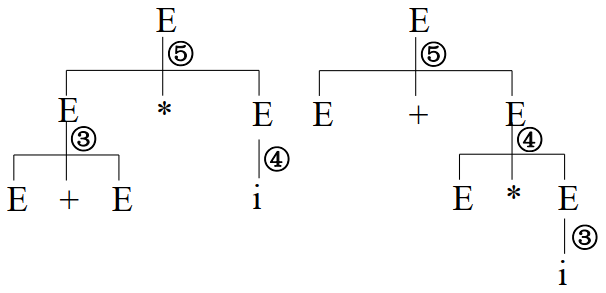如对句型E + E * i ，规范规约时句柄不唯一，所以整个规约过程就不唯一，编译所得的结果也将不唯一。

二、算符优先分析法的进一步讨论

1、算符优先文法（OPG）

1. 算符文法（OG）

若文法 G 中无形如 U → …VW… 的规则，V, W∈Vn，则称 G 为算符文法。

• 又称为OG文法。
• 理解：算符文法不允许两个非终结符相邻。

2. 优先关系

若G是一个OG文法， a, b ∈ Vt，U, V, W ∈ Vn

• ab ⇔ 文法中有形如 U → …ab…或 U → …aVb… 的规则。
• a ⋖ b ⇔ 文法中有形如 U → …aW… 的规则，其中 W ⇒ + \stackrel{+}{\Rightarrow} b… 或 W ⇒ + \stackrel{+}{\Rightarrow} Vb… 。
• a ⋗ b ⇔ 文法中有形如 U → …Wb… 的规则，其中 W ⇒ + \stackrel{+}{\Rightarrow} …a 或 W ⇒ + \stackrel{+}{\Rightarrow} …aV 。

例：文法G[E]

E → E + T | T
T → T * F | F
F → ( E ) | i

这里用<、>、= 来代替⋖、⋗、表示

对于 E → E + T：

∵ E ⇒ E + T ∴ + > +（规则3）

∵ T ⇒ T * F ∴ + < *（规则2）

∵ T ⇒ F ⇒ ( E ) ∴ + < (（规则2）

∵ T ⇒ F ⇒ i+ < i（规则2）

对于 F → ( E ) :

∵ E ⇒ E + T
( = )（规则1）、( < +（规则2）、+ > )（规则3）

3. 算符优先文法（OPG）

• OPG，Operator Precedence Grammar

定义：

对一OG文法，若在任意两个终结符之间，至多只有上述优先关系中的一种，则称该文法为算符优先文法（OPG）。

注：

• OG文法中运算是以中缀形式出现的。

• OPG文法 ⊆ OG文法（OPG文法不会出现两个非终结符相邻的句型）

• 算法语言中的表达式以及大部分语言成分的文法均是OG文法。

2、构造优先关系矩阵

•：若有U → …ab… 或 U → …aVb…，则 ab。

• ⋖：需要找出FIRSTVT 集

若文法有规则 W → …aU… ，对 ∀ b ∈ FIRSTVT(U)，有 a < b。

• ⋗：需要找出LASTVT 集

若文法有规则 W → …U b… ，对 ∀ a ∈ LASTVT(U)，有 a > b。

1. FIRSTVT 集

FIRSTVT(U) = { b | U ⇒ + \stackrel{+}{\Rightarrow} b… 或 U ⇒ + \stackrel{+}{\Rightarrow} Vb… , b∈ Vt , V∈ Vn }

构造FIRSTVT(U)的算法

1、若有规则 U → b… 或 U → Vb… ，则 b ∈ FIRSTVT(U)

• 定义的一步推导

2、若有规则 U → V… 且 b∈ FIRSTVT(V)，则 b∈ FIRSTVT(U)

• 定义的多步推导

注：因为V ⇒ + \stackrel{+}{\Rightarrow} b… 或 V ⇒ + \stackrel{+}{\Rightarrow} Wb…，所以有U ⇒ V… ⇒ + \stackrel{+}{\Rightarrow} b… 或 U ⇒ V… ⇒ + \stackrel{+}{\Rightarrow} Wb…

程序（伪代码）

设一个栈S和一个二维布尔数组F（行：Vn；列：Vt）
F[U, b] = TRUE iff b ∈ FIRSTVT(U)

PROCEDURE INSERT(U,b) // 申明一个过程，将符号对(U, b)插入S栈中
IF NOT F[U,b] THEN
BEGIN
F[U,b] := TRUE; // 首先置F[U,b]为真
并把(U,b)压入S栈
END;

main()
BEGIN
FOR 每个非终结符号U和终结符b DO
F[U,b] := FALSE; // 赋初值

FOR 每个形如 U → b... 或U → Vb... 的规则 DO
INSERT(U, b); // 完成算法的第1条

WHILE S栈非空 DO
BEGIN
把S栈的顶项弹出，记为(V,b) // b∈FIRSTVT(V)
FOR 每个形如U → V...的规则 DO
INSERT(U, b); // b∈FIRSTVT(U)
END // 完成算法的第2条
END

上述算法的工作结果是得到一个二维的布尔数组F，从F可以得到任何非终结符号 U 的 FIRSTVT：FIRSTVT(U) = { b | F[U, b] = TRUE }

2. LASTVT 集

LASTVT(U) = { a | U ⇒ + \stackrel{+}{\Rightarrow} …a 或 U ⇒ + \stackrel{+}{\Rightarrow} …aV, a∈ Vt , V∈ Vn }

构造LASTVT(U)的算法

1、若有规则 U → …a 或 U → …aV，则 a ∈ LASTVT(U)

• 定义的一步推导

2、若有规则 U → …V，且 a ∈ LASTVT(V)，则 a ∈ LASTVT(U)

• 定义的多步推导

函数实现（伪代码）

设一个栈 ST 和一个布尔数组 B
PROCEDURE INSERT(U, a)
IF NOT B[U, a] THEN
BEGIN
B[U, a] := TRUE；
把(U, a)推进ST栈；
END

main()
BEGIN
FOR 每个非终结符号U和终结符号a DO
B[U, a] := FALSE;

FOR 每个形如U → ...a 或 U → ...aV 的规则 DO
INSERT(U, a);

WHILE ST栈非空 DO
BEGIN
把ST栈的栈顶弹出，记为(V, a);
FOR 每条形如 U → ...V的规则 DO
INSERT(U, a);
END
END

3. 构造优先关系矩阵的算法

算法：

a, b∈Vt，V∈Vn

1、 从文法中找出形为 aVb 和 ab的部分，a = b。

2、从文法中找出形为aV的部分，对每个b∈FIRSTVT(V)，a < b

3、从文法中找出形为Va的部分，对每个b∈LASTVT(V)，b > a

代码：

FOR 每条规则U → x1 x2...xn DO
FOR i:=1 TO n-1 DO
BEGIN
IF xi和xi+1均为终结符, THEN
置 xi=xi+1
IF i≤n-2，且xi和xi+2都为终结符号但xi+1为非终结符号 THEN
置 xi=xi+2
IF xi为终结符号xi+1为非终结符号 THEN
FOR FIRSTVT(xi+1)中的每个b DO
置xi<b
IF xi为非终结符号xi+1为终结符号 THEN
FOR LASTVT(xi)中的每个a DO
置a>xi+1
END

例1：文法 G[E]

E → E + T | T
T → T * F | F
F → ( E ) | i

FIRSTVT+*()i
E
T
F
LASTVT+*()i
E
T
F
优先矩阵+*()i
+><<><
*>><><
(<<<=<
)>>>
i>>>

例2：文法 G[E]

E → E + E | E * E | ( E ) | i

• Vn = { E }

• Vt = { +, *, (, ), i }

FIRSTVT+*()i
E
LASTVT+*()i
E
优先矩阵+*()i
+<、><、><><
*<、><、><><
(<<<=<
)>>>
i>>>
• 产生冲突，该文法不属于算符优先文法

3、算符优先分析算法的设计

先定义优先级，在分析过程中通过比较相邻运算符之间的优先级来确定句型的“句柄”并进行归约。

1. 素短语

文法G的句型的素短语是一个短语，它至少包含一个终结符号，并且除它自身以外不再含有更小的素短语

例：有文法 G[E]

E → E + T | T
T → T * F | F
F → ( E ) | i

1）求句型 T + T * F + i 的最左素短语。
2）求句型 T + T + i 的最左素短语。

1）句型 T + T * F + i

语法树：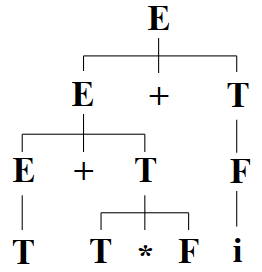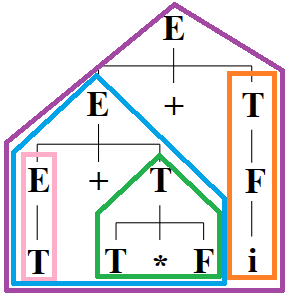短语是素短语？原因
T + T * F + i×包含其它短语T、T * F、i
T + T * F×包含其它短语T、T * F
T×不包含终结符
T * F
i

句柄：T
最左素短语：T * F

2）句型 T + T + i

语法树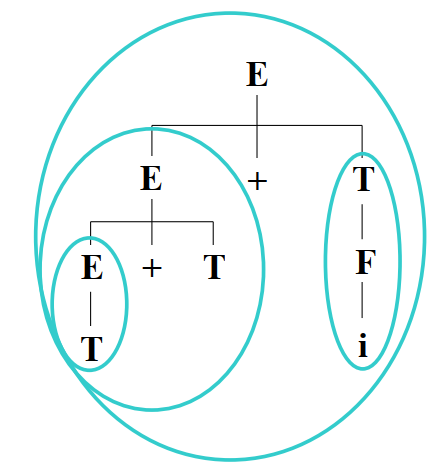短语是素短语？原因
T + T + i×包含其它短语T + T、i
T + T
T×不包含终结符
i

句柄：T
最左素短语：T + T

2. 确定当前句型的最左素短语

设有OPG文法句型：N1 a1 N2 a2 … Nn an Nn+1 #，其中Ni 为非终结符（可以为空）， ai为终结符。

定理

一个OPG句型的最左素短语是满足下列条件的最左子串：aj-1 Nj aj … Ni ai Ni+1 ai+1，其中 aj-1 < aj，aj = aj+1，aj+1 = aj+2，…，ai-2 = ai-1，ai-1 = ai，ai > ai+1

根据该定理，要找句型的最左素短语就是要找满足上述条件的最左子串。

注：

• 出现在 aj 左端和 ai 右端的非终结符号一定属于这个素短语，因为我们的运算是中缀形式给出的（OPG文法的特点）
N a N a N a N ⇒ N a W a N

例：文法G[E]，分析文法的句型 T + T * F + i。

E → E + T | T
T → T * F | F
F → ( E ) | i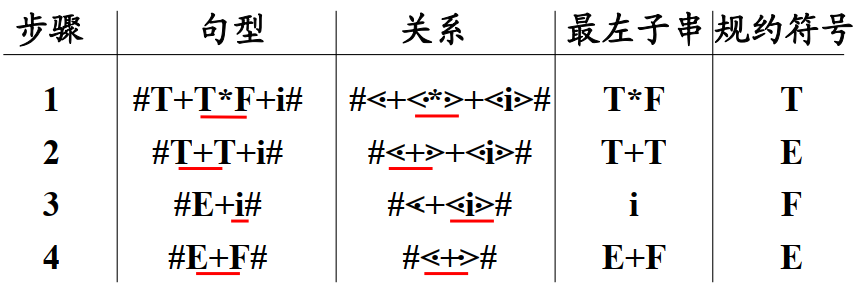可以看出：

• 每次规约的最左子串，确实是当前句型的最左素短语（从语法树可以看出来）
• 归约的不都是真正的句柄（仅 i 规约为 F 是句柄，但它同时也是最左素短语）
• 没有完全按规则进行规约，因为素短语不一定是简单短语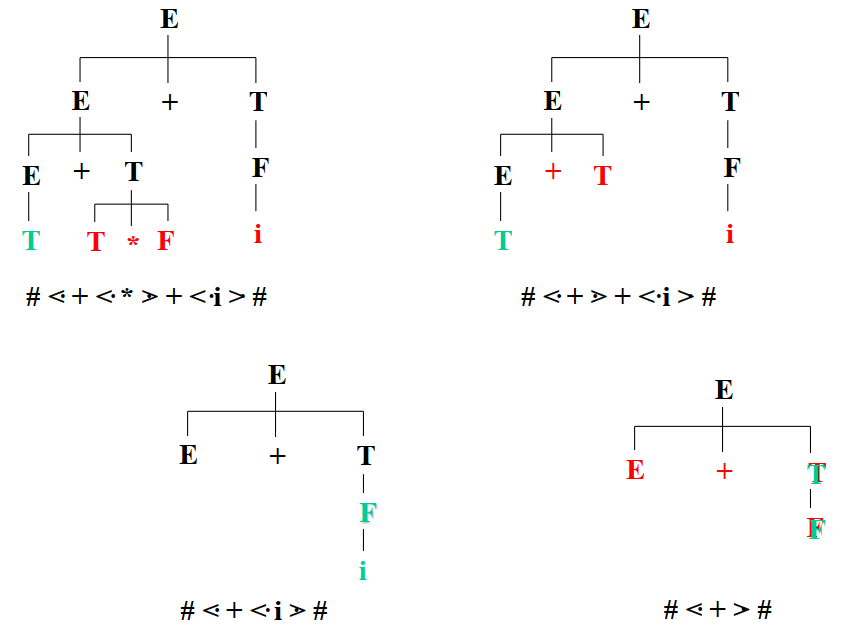3. 算符优先分析法的实现

基本部分是找句型的最左子串（最左素短语）并进行归约。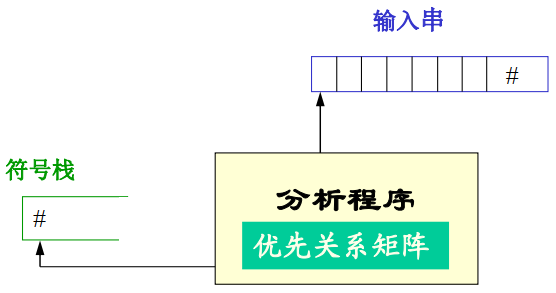• 当栈内终结符的优先级＜或＝栈外终结符的优先级时，移进；
• 当栈内终结符的优先级＞栈外终结符的优先级时，表明找到了素短语的尾，再往前找其头，并进行归约。

4、算符优先分析算法的局限性

由于算符优先分析并未对文法非终结符定义优先关系，所以就无法发现有单个非终结符组成的“可规约串”。

忽略非终结符在规约过程中的作用，存在某种危险性，可能导致把本来不成句子的输入串误认为是句子。

例：有文法 E → F + F，F → i

“i” 明显不是一个合法的句子。但按照下推自动机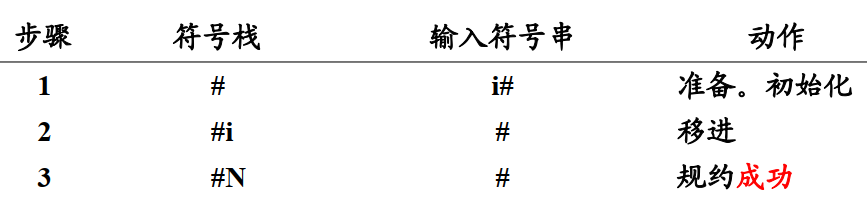展开全文编译原理
• 实验3《算符优先分析法设计与实现》 一、实验目的   加深对语法分析器工作过程的理解；加强对算符优先分析法实现语法分析程序的掌握；能够采用一种编程语言实现简单的语法分析程序；能够使用自己编写的分析程序对...

实验3《算符优先分析法设计与实现》

一、实验目的

加深对语法分析器工作过程的理解；加强对算符优先分析法实现语法分析程序的掌握；能够采用一种编程语言实现简单的语法分析程序；能够使用自己编写的分析程序对简单的程序段进行语法翻译。

二、实验内容

在实验1的基础上，用算符优先分析法编制语法分析程序，语法分析程序的实现可以采用任何一种编程语言和工具。

三、实验方法

1．实验采用C++程序语言进行设计，用户自定义输入文法进行分析；
2．开发工具为Visual Studio Code。

四、实验步骤

1．本程序中的文法，实质上是算数表达式的计算。
2．构建firstVT()和lastVT()这两个集合；
3．构建优先符号表；
4．构建词法分析的程序；
5．编写main函数，用户输入文法对语句利用算符优先文法进行判别。
6．算法的主体思想：
用栈存储已经看到的输入符号，用优先关系指导移动归约语法分析器的动作 ，如果栈顶的终结符和下一个输入符之间的优先关系是<或=，则语法分析器移动，表示还没有发现句柄的右端，如果是>关系，就调用归约。

五、实验结果
1. 运行实验代码，用户先输入文法个数，之后输入具体文法，程序根据输入进行FIRSTVT集、LASTVT集和算符优先表的计算，之后再对句子进行文法判别。
实验输入数据为：
6
S->#E#
P->i
F->P
T->F
E->T
E->E+T

测试结果：
测试结果符合预期结果，能够对句子i+i进行正确判别，用户也可以自定义其他语句进行实验。实验截图如下所示：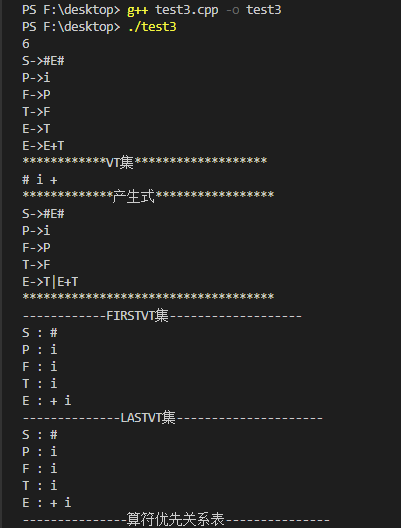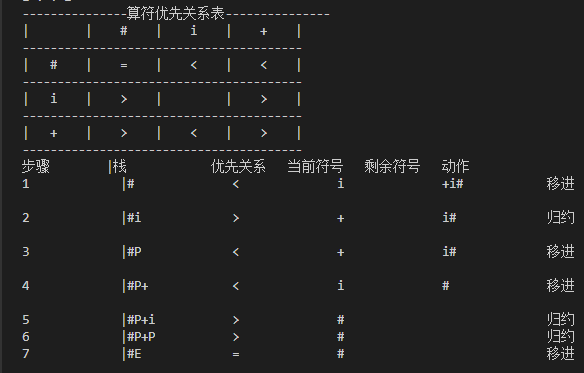六、实验结论

1．实验利用自定义的源程序进行测试，结果正确，符合预期结果，测试源码及结果截图和说明如上所示。
2．实验源代码如下所示：
test3.cpp

/**************************
Compiler Principle
test3 算符优先分析法设计与实现
author:zz
vs code
2019.05.05
***************************/
#include <iostream>
#include <cstring>
#include <cstdio>
#include <vector>
#include <stack>
#include <map>
#include <set>
#include <algorithm>
#include <string>
#include <cstdlib>
#include <cctype>
#define MAX 507

using namespace std;

class WF
{
public:
string left;
vector<string> right;
WF ( const string& str )
{
left = str;
}
void insert ( char str[] )
{
right.push_back(str);
}
void print ( )
{
printf ( "%s->%s" , left.c_str() , right.c_str() );
for ( int i = 1 ; i < right.size() ; i++ )
printf ( "|%s" , right[i].c_str() );
puts("");
}
};

char relation[MAX][MAX];
vector<char> VT;
vector<WF> VN_set;
map<string,int> VN_dic;
set<char> first[MAX];
set<char> last[MAX];
int used[MAX];
int vis[MAX];

void dfs (  int x )
{
if ( vis[x] ) return;
vis[x] = 1;
string& left = VN_set[x].left;
for ( int i = 0 ; i < VN_set[x].right.size() ; i++ )
{
string& str = VN_set[x].right[i];
if ( isupper(str) )
{
int y = VN_dic[str.substr(0,1)]-1;
if ( str.length() > 1 && !isupper(str ) )
first[x].insert ( str );
dfs ( y );
set<char>::iterator it = first[y].begin();
for ( ; it!= first[y].end() ; it++ )
first[x].insert ( *it );
}
else
first[x].insert ( str );
}
}

void make_first ( )
{
memset ( vis , 0 , sizeof ( vis ) );
for ( int i = 0 ; i < VN_set.size() ; i++ )
if ( vis[i] ) continue;
else dfs ( i );
#define DEBUG
#ifdef DEBUG
puts("------------FIRSTVT集-------------------");
for ( int i = 0 ; i < VN_set.size() ; i++ )
{
printf ( "%s : " , VN_set[i].left.c_str() );
set<char>::iterator it = first[i].begin();
for ( ; it!= first[i].end() ; it++ )
printf ( "%c " , *it );
puts ("" );
}
#endif
}

void dfs1 ( int x )
{
if ( vis[x] ) return;
vis[x] = 1;
string& left = VN_set[x].left;
for ( int i = 0 ; i < VN_set[x].right.size() ; i++ )
{
string& str = VN_set[x].right[i];
int n = str.length() -1;
if ( isupper(str[n] ) )
{
int y = VN_dic[str.substr(n,1)]-1;
if ( str.length() > 1 && !isupper(str[n-1]) )
last[x].insert ( str );
dfs1 ( y );
set<char>::iterator it = last[y].begin();
for ( ; it != last[y].end() ; it++ )
last[x].insert ( *it );
}
else
last[x].insert ( str[n] );
}
}

void make_last ( )
{
memset ( vis , 0 , sizeof ( vis ) );
for ( int i = 0 ; i < VN_set.size() ; i++ )
if ( vis[i] ) continue;
else dfs1 ( i );
#define DEBUG
#ifdef DEBUG
puts("--------------LASTVT集---------------------");
for ( int i = 0 ; i < VN_set.size() ; i++ )
{
printf ( "%s : " , VN_set[i].left.c_str() );
set<char>::iterator it = last[i].begin();
for ( ; it!= last[i].end() ; it++ )
printf ( "%c " , *it );
puts ("" );
}
#endif
}

void make_table ( )
{
for ( int i = 0 ; i < MAX ; i++ )
for ( int j = 0 ; j < MAX ; j++ )
relation[i][j] = ' ';
for ( int i = 0 ; i < VN_set.size() ; i++ )
for ( int j = 0 ; j < VN_set[i].right.size() ; j++ )
{
string& str = VN_set[i].right[j];
for ( int k = 0 ; k < str.length()-1 ; k++ )
{
if ( !isupper(str[k]) && !isupper(str[k+1]) )
relation[str[k]][str[k+1]] = '=';
if ( !isupper(str[k]) && isupper(str[k+1]) )
{
int x = VN_dic[str.substr(k+1,1)]-1;
set<char>::iterator it = first[x].begin();
for ( ; it != first[x].end() ; it++ )
relation[str[k]][*it] = '<';
}
if ( isupper(str[k]) && !isupper(str[k+1]) )
{
int x = VN_dic[str.substr(k,1)]-1;
set<char>::iterator it = last[x].begin();
for ( ; it != last[x].end() ; it++ )
relation[*it][str[k+1]] = '>';
}
if ( k > str.length()-2 ) continue;
if ( !isupper(str[k]) && !isupper(str[k+2]) && isupper(str[k+1]) )
relation[str[k]][str[k+2]] = '=';
}
}
#define DEBUG
#ifdef DEBUG
for ( int i = 0 ; i < VT.size()*5 ; i++ )
printf ("-");
printf ( "算符优先关系表" );
for ( int i = 0 ; i < VT.size()*5 ; i++ )
printf ( "-" );
puts("");
printf ( "|%8s|" , "" );
for ( int i = 0 ; i < VT.size() ; i++ )
printf ( "%5c%5s" , VT[i] , "|" );
puts ("");
for ( int i = 0 ; i < (VT.size()+1)*10 ; i++ )
printf ("-");
puts("");
for ( int i = 0 ; i < VT.size() ; i++ )
{
printf ( "|%4c%5s" , VT[i] , "|");
for ( int j = 0 ; j < VT.size() ; j++ )
printf ( "%5c%5s" , relation[VT[i]][VT[j]] , "|" );
puts ("");
for ( int i = 0 ; i < (VT.size()+1)*10 ; i++ )
printf ("-");
puts("");
}
#endif
}

int fa[MAX];

int _find ( int x  )
{
return x==fa[x]?x:fa[x] = _find ( fa[x] );
}

bool judge ( char x , char y )
{
if ( _find ( x ) == _find ( y ) )
return true;
return false;
}

void _union ( char x , char y )
{
x = _find(x);
y = _find(y);
fa[x] = y;
}

void print ( string s1 , string s2 , string s3 , string s4 , string s5 , string s6 )
{
printf ( "%-14s|%-15s%-15s%-15s%-15s%-15s\n" , s1.c_str(), s2.c_str(), s3.c_str() ,s4.c_str(),s5.c_str() , s6.c_str() );
}

void init ( )
{
for ( int i = 0 ; i < MAX ; i++ )
fa[i] = i;
for ( int i = 0 ; i < VN_set.size() ; i++ )
{
string& left = VN_set[i].left;
for ( int j = 0 ; j < VN_set[i].right.size() ; j++ )
{
string& str = VN_set[i].right[j];
if ( left.length() == 1 && str.length() == 1 )
{
// cout << left << " " << str << endl;
_union ( left , str );
// cout << _find(left) << " " << _find ( str )  << endl;
}
}
}
print("步骤","栈","优先关系","当前符号","剩余符号","动作");
}

string get_stk ( vector<char>& stk )
{
string ret = "";
for ( int i = 0 ; i < stk.size() ; i++ )
ret += stk[i];
return ret;
}

bool check ( const string& str1 , const string& str2 )
{
if ( str1.length() != str2.length() )
return false;
for ( int i = 0 ; i < str1.length() ; i++ )
if ( isupper(str1[i]) )
{
if ( !judge(str1[i],str2[i])) return false;
}
else
{
if ( str1[i] != str2[i] ) return false;
}
return true;
}

string reduction ( string src )
{
for ( int i = 0 ; i < VN_set.size() ; i++ )
for ( int j = 0 ; j < VN_set[i].right.size() ; j++ )
if ( check ( VN_set[i].right[j] , src ) )
return VN_set[i].left;
return "";
}

void move_reduction ( string src )
{
init ();
//cout <<"flag : " << check ( "P+P" , "E+T" ) << endl;;
vector<char> stk;
int steps= 1;
src += "#";
stk.push_back ( '#' );
for ( int i = 0 ; i < src.length() ; i++ )
{
char top = stk[stk.size()-1];
for ( int j = stk.size()-1 ; j >= 0 ; j-- )
if ( isupper(stk[j]) ) continue;
else
{
top = stk[j];
break;
}
char ch = relation[top][src[i]];
if ( ch == '<' || ch == '=' )
{
string temp = "";
if ( i == src.length() - 1 )
print ( temp+(char)(steps+48) , get_stk( stk ) , temp+ch , temp+src[i] , "" , "移进" );
else
print ( temp+(char)(steps+48) , get_stk( stk ) , temp+ch , temp+src[i] , src.substr(i+1,src.length()-i-1) , "移进" );
stk.push_back ( src[i] );
}
else
{
string temp ="";
string str ="";
int x = stk.size()-2;
if ( i == src.length() )
print ( temp+(char)(steps+48) , get_stk(stk) , temp+ch , temp + src[i] , "" , "归约" );
else
print ( temp+(char)(steps+48) , get_stk(stk) , temp+ch , temp + src[i] , src.substr(i+1,src.length()-i-1) , "归约" );
while ( true )
{
if ( stk.size() == 0 ) break;
if ( !isupper(stk[x] ) &&relation[stk[x]][top] == '<' )
break;
x--;
}
for ( int j = stk.size()-1 ; j > x; j-- )
{
str += stk[j];
stk.pop_back();
}
//cout << "YES : " << i <<" " << str <<  endl;
str = reduction(str);
//cout << "finish: " << i << " " << str << endl;
for ( int j = 0 ; j < str.length() ; j++ )
stk.push_back ( str[j] );
/*if ( i == src.length() )
print ( temp+(char)(steps+48) , get_stk(stk) , temp+ch , temp + src[i] , "" , "移进" );
else
print ( temp+(char)(steps+48) , get_stk(stk) , temp+ch , temp + src[i] , src.substr(i+1,src.length()-i-1) , "移进" );
stk.push_back ( src[i] );*/
i--;
}
steps++;
}
//string temp ="";
//cout << "result" << endl;
//print ( temp+(char)(steps+48) , get_stk(stk) , "","","","");
}

int main ( )
{
int n;
char s[MAX];
while ( ~scanf ( "%d" , &n ) )
{
memset ( used , 0 , sizeof ( used ) );
for ( int i = 0 ; i < n ; i++ )
{
scanf ( "%s" , s );
int len = strlen(s),j;
for ( j = 0 ; j < len ; j++ )
if ( s[j] == '-' )
break;
s[j] = 0;
if ( !VN_dic[s] )
{
VN_set.push_back ( WF(s) );
VN_dic[s] = VN_set.size();
}
int x = VN_dic[s]-1;
VN_set[x].insert ( s+j+2 );
for ( int k = 0 ; k < j; k++ )
if ( !isupper(s[k] ) )
{
if ( used[s[k]] ) continue;
used[s[k]] = 1;
VT.push_back ( s[k] );
}
for ( int k = j+2 ; k < len; k++ )
if ( !isupper(s[k] ) )
{
if ( used[s[k]] ) continue;
VT.push_back ( s[k] );
used[s[k]] = VT.size();
}
}
#define DEBUG
#ifdef DEBUG
puts ("************VT集*******************");
for ( int i = 0 ; i < VT.size() ; i++ )
printf ( "%c " , VT[i] );
puts ("");
puts("*************产生式*****************");
for ( int i = 0 ; i < VN_set.size() ; i++ )
VN_set[i].print();
puts("************************************");
#endif
make_first();
make_last();
make_table();
move_reduction("i+i");
}
}

七、实验小结
1. 本次实验通过对文法进行处理，获取FIRSTVT()集合、LASTVT()集合和算符优先关系表，从而进一步对语句进行分析判断。通过本次实验我对语义分析有了更进一步的了解。
2. 实验过程中遇到的问题有：
①FIRSTVT()和LASTVT()这两个集合的构建。
解决方式：对书本中两个集合进一步优先，写出伪代码，进行实现。
②如何利用FIRSTVT（）和LASTVT（）集合构建算符优先关系表。
解决方式：多次尝试可得如下伪代码，从而实现该关系表的代码化。
FOR 每个产生式 P->X1 X2 ……Xn
DO  FOR  i:=1  TO   n-1    DO
IF  X[i]和X[i+1]均为终结符
THEN   置 X[i]=X[i+1]
IF  X[i]和X[i+2]均为终结符,X[i+1]为非终结符,i≤n-2,
THEN   置 X[i]=X[i+2]
IF  X[i]为终结符, 但X[i+1]为非终结符
THEN  FOR  FIRSTVT(X[i+1])中的每个a
DO  置 X[i]<a
IF  Xi为非终结符, 但X i+1 为终结符
THEN  FOR  LASTVT(X i )中的每个a
DO   置 a>X[i+1]

1. 实验的输入输出格式可进一步调整优化，代码的注释有待增加，可读性有进一步提升空间。
展开全文• 以陈火旺的《编译原理》里描述的为基础，自己写的一个程序
• 自底向上分析之算符优先分析法说明：以老师PPT为标准，借鉴部分教材内容，AlvinZH学习笔记。基本过程1. 一般方法：采用自左向右地扫描和分析输入串，从输入符号串开始，通过反复查找当前句型的句柄(最左简单短语)，...
• 算符优先分析法-java实现

千次阅读 多人点赞 2019-12-03 17:50:33
算符优先分析器-java实现 一、判断是否是算符文法 算符文法定义:一个文法，如果它的任一产生式的右部都不含两个相继(并列)的非终结符，即不含如下形式的产生式右部:…QR…,则称该文法为算符文法 二、求Firstvt和...算符优先分析器 java
• （Python实现，注释详细）直接输入：3+4*5，一般的...(1)第一阶段，运用算符优先分析算法完成计算器中对算术表达式的语法分析； (2)第二阶段，设计属性文法，改造第一阶段的程序，完成算术表达式的计算和相关的输出。
• 算符优先分析法是一种简单且直观的自下而上分析方法，它特别适合于分析程序语言中的各类表达式，并且宜于手工实现。所谓算符优先分析，就是依照算术表达式的四则运算过程来进行语法分析，即这种分析方法要预先规定...编译器
• 算符优先分析法-思路方法在这里

千次阅读 多人点赞 2020-05-16 20:48:28
编写一个算符优先分析程序，能实现以下功能： 1.输入文法，判断是否为算符文法； 2.构造并输出该文法的每个非终结符的 FIRSTVT 集和 LASTVT 集； 3.构造并输出算符优先分析表，判断是否为算符优先文法，若不是提示...编程语言 算法
• 实验三 算符优先分析算法的设计与实现 8 学时) 一 实验目的 根据算符优先分析法 对表达式进行语法分析 使其能够判断一个表达式是否正确 过算符优先分析方法的实现加深对自下而上语法分析方法的理解 二 实验要求 1...
• 算符优先分析法研究--附源程序目 录1 课程设计的目的和要求21.1 课程设计的目的21.2 课程设计的要求22 系统描述22.1 自底向上分析方法的描述:22.2 算符优先文法的描述：23)输入符号串，进行移进-规约分析。...
• ONLYAccelerator/编译原理算符优先分析法https://gitee.com/q443808626/Compilationprinciple2.git 垃圾代码，仅供分享！
• java实现算符优先分析法

热门讨论 2010-06-04 14:00:29Java 算符优先
• 算符优先分析法是一种自底向上的语法分析方法。在算符文法中，任何一个规则右部都不存在两个非终结符相邻的情况。通过比较两个相继出现的终结符a，b的优先级，然后借助优先级关系，来确定句型的可归约串并进行归约。...
• 编译原理：算符优先分析法

千次阅读 2020-05-28 08:22:45
• 先乘除，再加减。算符的优先关系是确定好的，根据文法来说是一定的（这保证了归约是按着推导的过程来的）（由终结符优先关系定义可知，越早需要归约的串他的优先级越高）。...这就是算法优先分析方法的原理 ...编译器
• 算符优先分析法的简单实现

千次阅读 2018-05-11 19:05:50
算符优先分析法即是一种针对算符优先文法的分析方法。 二.如果一个文法的任一产生式的右部都不存在两个相邻的非终结符，则称这个文法为算符文法(OG)。 三.假定文法G是一个不含e的算符文法，a,b∈Vt，P,Q,R∈Vn，...
• 例子：该例子使用的是简单优先分析法 这种方式的问题，只有在有树图的情况下才好判断句柄，也就是难以寻找句柄，对于电脑来说，不容易实现，且耗费时间多 相邻文法符号之间的优先关系： 句柄寻找： 句柄的概念：...编译器
• 算符优先分析方法设计一个分析解释程序，对输入的赋值语句、输出语句、清除语句进行词法分析、语法分析、表达式求值并存储于指定变量中；若存在错误，提示错误相关信息。
• 编译原理 —— 算符优先分析法

万次阅读 多人点赞 2019-05-17 17:12:36
什么是算符优先分析法 算符优先分析法是一种简单、直观的自下而上分析法 算符优先分析法就是仿照算术表达式的四则运算过程而设计的一种语法分析方法。 这种分析方法首先要规定运算符之间（确切地说终结符之间）的...
• 这是一个用c++语言实现的编译原理里面实现算符优先分析法的程序，包括创建FIRSTVT，LASTVT，和分析表。
• 算符优先分析文法是一种工具，在编译的过程中，隶属于语法分析环节，却又与中间代码的生成息息相关，编译可以分为五个阶段：词法分析、语法分析、语义分析（中间代码的生成）、代码优化、目标代码生成。语法分析是指...
• 编译原理 算符优先文法 实验报告 代码 运行成功////////////
•  算符优先分析法(Operator Precedence Parse)是仿效四则运算的计算过程而构造的一种语法分析方法。算符优先分析法的关键是比较两个相继出现的终结符的优先级而决定应采取的动作。  优点：简单，有效，适合表达式......